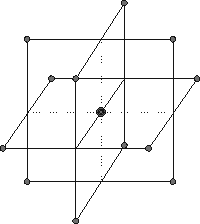# Box-Behnken Design¶Above is a representation of a classic Box-Behnken (BB) design for 3 factors.

Numeric Factors: How many continuous numeric factors are involved in the experiment?

Categoric Factors: How may discrete or categoric factors are involved in the experiment?

Note

For BB’s the number of runs required for the numeric factors will be multiplied by the number of categoric combinations. If two categoric factors having three levels each are added to this design the number of runs will be multiplied by nine. Consider optimal designs when you have both numeric and categoric factors.

Blocks: Some Box-Behnken designs can be blocked. The number of blocks depends on the number of factors. If you need blocks in your design and the BB design cannot do what you need, switch to an optimal design.

Center Points: By default there will be some center points in a BB design, the number varies somewhat with the number of factors and blocks. Adding more center points is okay. Removing center points will adversely affect the design’s precision capability.